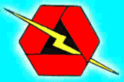rexresearch.com

Gabor DOMOKOS & Peter VARKONYI

Gömböchttp://www.nytimes.com/pages/magazine/index.html
The New York Times
December 9, 2007

The Self-Righting Object

by Clive Thompson

The Gomboc is a roundish piece of clear synthetic material with gently peaked, organic curves. It looks like a piece of modern art. But if you tip it over, something unusual happens: it rights itself.

The Gomboc is the physical realization of a mathematical theorem: that a "mono-monostatic" object -- one that has a single stable point of equilibrium, or balance -- must exist. And so it does. No matter how you orient it, the Gomboc always rights itself.

It leans off to one side, rocks to and fro as if gathering strength and then, presto, tips itself back into a “standing” position as if by magic. It doesn’t have a hidden counterweight inside that helps it perform this trick, like an inflatable punching-bag doll that uses ballast to bob upright after you whack it. No, the Gomboc is something new: the world’s first self-righting object.

The Gomboc is a result of a long mathematical quest. In 1995, the Russian mathematician Vladimir Arnold mused that it would be possible to create a “mono-monostatic” object — a three-dimensional thingy that purely by dint of its geometry had only one possible way to balance upright.

The challenge intrigued two scientists — Gabor Domokos and Peter Varkonyi, both of the Budapest University of Technology and Economics. They spent a few years doing the math, and it seemed as if a mono-monostatic object could, in fact, exist. They began looking to see if they could find a naturally occurring example; at one point, Domokos was so obsessed that he spent hours testing 2,000 pebbles on a beach to see if they could right themselves. (None could.)

After several more years of scratching their heads, they finally hit upon a shape that looked promising. They designed it on a computer, and when it came back from the manufacturer, they nervously tipped it over, wondering if all their work would be for naught. Nope: the Gomboc performed perfectly. “It’s a very nice mathematical problem because you can hold the proof in your hands — and it’s quite beautiful,” Varkonyi says.

Yet the scientists now say that Mother Nature may have beaten them in the race after all. They have noticed that the Gomboc closely resembles the shell of a tortoise or a beetle, creatures whose round-shelled backs help them right themselves when flipped over. “We discovered it with mathematics,” Domokos notes, “but evolution got there first.”

What is Gömböc?

Gömböc (pronounced as 'goemboets')?

The 'Gömböc' is the first known homogenous object with one stable and one unstable equilibrium point, thus two equilibria altogether on a horizontal surface. It can be proven that no object with less than two equilibria exists.The stable equilibrium (S)

If placed on a horizontal surface in an arbitrary position the Gömböc returns to the stable equilibrium point, similar to 'weeble' toys. While the weebles rely on a weight in the bottom, the Gömböc consists of homogenous material, thus the shape itself accounts for self-righting.

The unstable equilibrium (I)

The single unstable equilibrium point of the Gömböc is on the opposite side. It is possible to balance the body in this position, however the slightest disturbance makes it fall, similar to a pencil balanced on its tip.

The question whether Gömböc-type objects exist or not was posed by the great Russian mathematician V. I. Arnold at a conference in 1995, in a conversation with Gabor Domokos.

Mathematical background

Convexity and homogeneity are crucial properties of Gömböc. Weebles are straightforward examples of inhomogenous objects with Gömböc-type behaviour. Similarly, it is easy to create homogenous but concave Gömböc-like forms due to the fact that concave bodies cannot roll on all points of their circumference.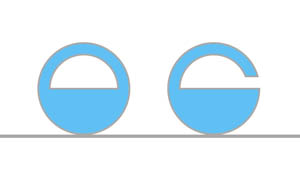Concave Gömböc-type planar shapes.

Shapes with a unique stable equilibrium are called monostatic; those with only one additional unstable point are referred to as mono-monostatic. Thus the Gömböc is the first convex, homogenous, mono-monostatic object.

Planar Gömböc

All planar, convex shapes can be defined by a function R(a) in a polar coordinate system with origin at the center of gravity of the object (G). On horizontal surfaces, all objects start rolling in a way that sends G lower, i.e. such that R decreases at the point of contact with the underlying surface. Equilibria occur if dR/da = 0 at this point. A balance point is stable at minima of R (d2R/da2 > 0) and unstable at maxima (d2R/da2 < 0). Minima of R are followed by maxima and vice versa, thus the numbers of stable and unstable equilibria are equal. In addition, the following interesting statement can be proven:

Theorem 1.:
All planar, convex, homogenous shapes have at least 2 stable and 2 unstable equilibria.

If an object had only one equilibrium point of each type, the diagram of the corresponding function R(a) would have just one maximum and one minimum. In this case one could cut it by a horizontal line R = R0 such that the two parts R > R0 and R < R0 of the function have equal (length p) horizontal projection. This would correspond cutting the original object to a thin (R < R0) and a thick (R > R0) part by a line crossing the center of gravity G. Imagine supporting the planar object along this line. In order to maintain moment balance, G should be off the line, on the thick side, which is contradicts our previous statement that G is on the line. Thus we arrived at a contradiction and therefore Theorem 1 is true.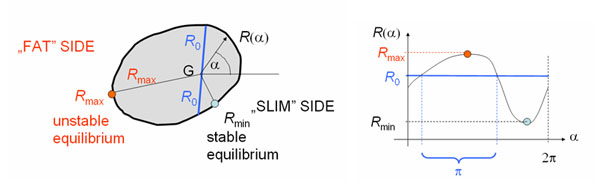The diagram R(a) diagram (left panel) and the corresponding body (right panel).

As we have just proven, there is no planar, Gömböc-type object. This surprisingly simple fact is the physical analogue of a classical mathematical theorem:

Four vertex theorem:
The curvature of a simple closed planar curve has at least four local extrema.

There are numerous generalizations of the Four vertex theorem as well as many related theorems in geometry, which are sometimes called Four vertex theorems together. If there were no Gömböc in 3D, this fact would be an additional member of the Four vertex theorem family.

Basic idea of the Gömböc

Similar to planar objects, 3D shapes can be defined by a function R(j,q) in a spherical coordinate system around their centers of gravity.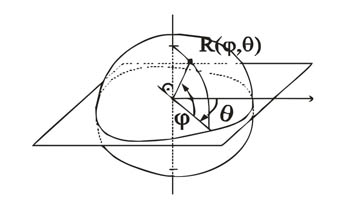Definition of a 3D shape in spherical coordinate system.

Local minima and maxima of R again correspond to stable and unstable equilibria, but the object has additional balance points at saddles of R. According to the Poincaré-Hopf theorem the number of equilibria (s, u, t, respectively) of the three types satisfies s + u - t = 2 for all objects isomorphic to spheres. One could imagine three analogues of Theorem 1 (stating s>1 and u>1 for planar objects):

* a) s > 1,
* b) u > 1,
* c) s + u> 2,

however a) and b) are easy to confute:

s > 1 is not true as shown by this counterexample, for which s = t = 1, u = 2: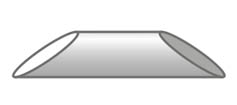There are simple counterexamples for i > 1, too. In this case, u = t = 1, s = 2: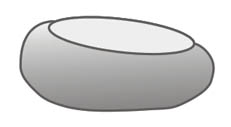The third possibility is the question of the Gömböc itself: are there 3D convex, homogenous bodies with s = u = 1 (thus t = 0)? We can try to extend the planar proof to show the nonexistence of such bodies. If there was such a shape, the corresponding function R(j,q) would have only one minimum and one maximum. The surface of the body could be cut by a level set R = R0 to a thin and a thick part of equal size (ie. the spatial angles determined by the two parts from G are of equal sizes). If this level set is a planar curve (i.e. a circle), we get to contradiction, similar to the 2D case. However, it can also be spatial curve, as, for example, the curve on tennis balls. In this case, the separation of the body to an upper thick and a lower thin part does not mean that G has to be in the upper part. Thus, the planar proof does not apply in 3D.The line separating the thick (yellow) and thin (green) parts of a hypothetical mono-monostatic body can be, but is not necessarily planar.

The ‘failure' of the proof yields some idea for the shape of a spatial Gömböc. This idea was used to construct a two-parameter closed formula, for which it was analytically proven that appropriate parameter values result in an object with s = u = 1. Unfortunately due to the additional constraint of convexity, the constructed form was almost identical to a simple sphere. Thus, this construction verified the existence of the Gömböc theoretically, but the existence of characteristic (visually obvious) mono-monostatic forms was still a question.Some members of the two-parameter family of bodies used in the analytical proof.

The ‘real‘ Gömböc

The ‘theoretical' proof raised the question: why did we fail to get a characteristic shape? Either the formula constructed for the proof was not good enough or some deeper reason was hiding behind the failure. The fact that Gömböc-type shapes proved to bear similar features to spheres and the lack of such shapes in a sample of 2000 pebbles at the island of Rhodes both suggested, that forms “far away" from the sphere can not have s = i = 1. Nevertheless, using a different approach the real Gömböc could be constructed. The form presented below is based on the idea of the tennis-ball. It consists of segments of simple surfaces (cylinder, ellipsoid, cone) and planes. The new shape is obviously convex. Numerical integration reveals that its center of gravity is slightly below the origin; this fact makes it easy to show that it is mono-monostatic.

Of course, infinite number of shapes have these properties, the figures show one of these. The fabricated Gömböc models are also slightly different: they consist of more segments, which makes the stability properties of the equilibria more robust and the dynamical behavior of the rolling objects more intuitive.Simple segments are connected together to construct the GömböcThe R=constant level curves of the Gömböc show clearly the tennis ball-shape.

Related publications:

 G. Domokos, J. Papadopulos, A. Ruina: Static equilibria of planar, rigid bodies: Is there anything new? Journal of Elasticity 36 pp. 59-66, 1994.

 P.L. Várkonyi, G. Domokos: Static equilibria of rigid bodies: dice, pebbles and the Poincare-Hopf Theorem. J. Nonlinear Sci. Vol 16: pp 255-281, 2006.

 G. Domokos: My lunch with Arnold. Mathematical Intelligencer 28 (4) pp. 31-33, 2006.

 P.L. Várkonyi, G. Domokos: Mono-monostatic bodies: the answer to Arnold's question Mathematical Intelligencer 28 (4) pp34-38 (2006)

VideosGomboc: How Turtles Self-RightGömböc - Hungarian Invention

Gömböc

A Gömböc ( pronounced [Gœmbœts] ) is a convex three-dimensional homogeneous body which, when resting on a flat surface, has just one stable and one unstable point of equilibrium. To avoid having two stable equilibria, the body must have minimal "flatness", and at the same time, to avoid having two unstable equilibria, it must also have minimal "thinness". This shape represents a class (i.e., it is not unique); the shape has very fine tolerances, outside which it is no longer mono-monostatic.

It is conjectured that there also exist convex polyhedra with just one stable face and one unstable point of equilibrium. The minimum number of faces could be large.

The Gömböc mimics the "self-righting" abilities of shelled animals such as turtles and beetles. Such a shape was conjectured by Russian mathematician Vladimir Arnold as a mono-monostatic body.

The shape was developed by Gábor Domokos (head of Mechanics, Materials and Structures at Budapest University of Technology and Economics in Hungary) and a former student of his, Péter Várkonyi (at Princeton University). The Gömböc made the front page of mathematical journal The Mathematical Intelligencer.

Domokos and his wife Réka developed a classification system for shapes based on their points of equilibrium by collecting pebbles from a beach and noting their equilibrium points. The Gömböc was developed in conjunction with that system as a supposed "perfect" self-righting mechanism.

Gömb in Hungarian means "sphere", and gömböc refers to a sphere-like object. (It is mostly known in the folk culture as kis gömböc, a round creature in the loft that remained from a killed pig, which swallows everyone one after the other who goes to see what happened to the previous ones.) The mathematical Gömböc has indeed sphere-like properties. In particular its flatness and thinness are minimal, and this is the only type of nondegenerate object with this property. The sphere has also minimal flatness and thinness, however, it is degenerate.(cf. Várkonyi & Domokos, 2006.)

On 13 February 2009 Domokos appeared on the British television programme QI where he explained how the gömböc works.

References

1. CBC Quirks and Quarks 2007-10-27: "Turning Turtles". Interview with Dr. Gabor Domokos.
2. Varkonyi, P.L., Domokos, G.: Mono-monostatic bodies: the answer to Arnold's question. The Mathematical Intelligencer, 28 (4) pp 34–38.(2006.)
3. Gergely, Andras: Boffins develop a 'new shape' called Gomboc, The Age (via Reuters), February 13, 2007.
4. A kis gömböc, folk tale in HungarianYour Support Maintains this Service --